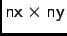# OOF: Finite Element Analysis of Microstructures

/initialize/uniformNext: /initialize/uniform/isotropic, etc. Up: The Menus Previous: /log/save   Contents

# /initialize/uniform

This menu creates a uniform rectangular grid (finite element mesh) of a single type of element. Variables in the menu determine the shape of the rectangle, how it is divided into finite elements, and what type of nodes to use. The grid is then initialized by executing a function representing one of the element types. After a uniform grid is created, the grid can be made inhomogeneous by selecting elements (section 3.19) and replacing them (section 3.3).

The size of the grid is determined by the variables height and width. The grid is divided intorectangular elements, each of which is then divided into two triangular elements according to the variable diagonals.

 Menus Functions Variables empty nx = 20 isotropic ny = 20 cubic width = 1 hexagonal height = 1 orthorhombic diagonals = liberal eds_el interior_nodes = xy damisotropic boundary_nodes = xy damage etc.

SubsectionsNext: /initialize/uniform/isotropic, etc. Up: The Menus Previous: /log/save   Contents
/* Send mail to the OOF Team *//* Go to the OOF Home Page */